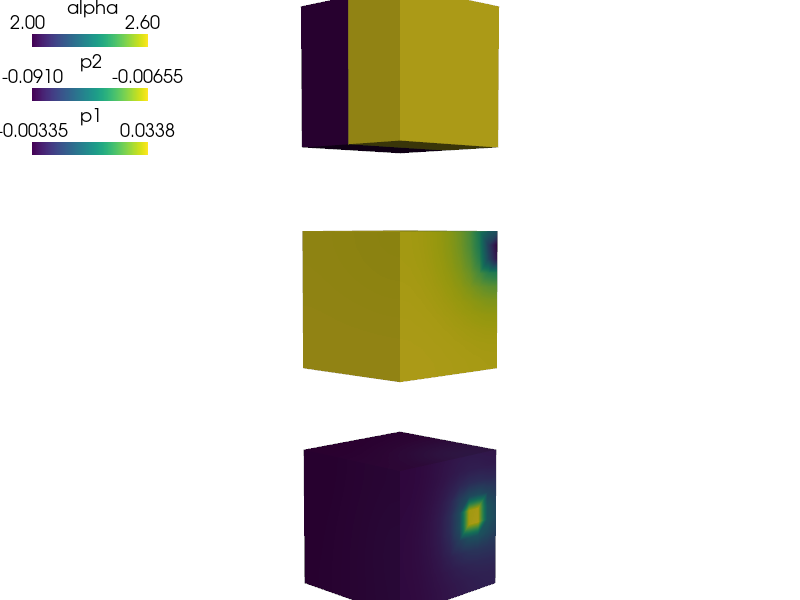# diffusion/darcy_flow_multicomp.py¶

Description

Each of the two equations describes a flow in one compartment of a porous medium. The equations are based on the Darcy flow and the i-th compartment is defined in.whereis the local permeability of the i-th compartment,is the perfusion coefficient related to the compartmentsand,are sources or sinks which represent the external flow into the i-th compartment andis the pressure in the i-th compartment.source code

r"""
Each of the two equations describes a flow in one compartment of a porous
medium. The equations are based on the Darcy flow and the i-th compartment is
defined in :math:\Omega_{i}.

.. math::
\int_{\Omega_{i}} K^{i} \nabla p^{i} \cdot \nabla q^{i}+\int_{\Omega_{i}}
\sum_{j} \bar{G}\alpha_{k} \left( p^{i}-p^{j} \right)q^{i}
= \int_{\Omega_{i}} f^{i} q^{i},
.. math::

where :math:K^{i} is the local permeability of the i-th compartment,
:math:\bar{G}\alpha_{k} = G^{i}_{j} is the perfusion coefficient
related to the compartments :math:i and :math:j, :math:f^i are
sources or sinks which represent the external flow into the i-th
compartment and :math:p^{i} is the pressure in the i-th compartment.
"""

from __future__ import absolute_import
from sfepy.base.base import Struct
import numpy as nm
from sfepy import data_dir

filename_mesh = data_dir + '/meshes/3d/cube_medium_hexa.mesh'
G_bar = 2.0
alpha1 = 1.3
alpha2 = 1.0

materials = {
'mat': ('mat_fun')
}

regions = {
'Omega': 'cells of group 0',
'Sigma_1': ('vertex 0', 'vertex'),
'Omega1': ('copy r.Omega', 'cell', 'Omega'),
'Omega2': ('copy r.Omega', 'cell', 'Omega'),
'Source': 'cell 24',
'Sink': 'cell 1',
}

fields = {
'pressure':('real', 1, 'Omega', 1)
}

variables = {
'p1': ('unknown field', 'pressure'),
'q1': ('test field', 'pressure', 'p1'),
'p2': ('unknown field', 'pressure'),
'q2': ('test field', 'pressure', 'p2'),
}

ebcs = {
'P1': ('Sigma_1', {'p1.0' : 0.0}),
}

equations = {
'komp1': """dw_diffusion.5.Omega1(mat.K, q1, p1)
+ dw_volume_dot.5.Omega1(mat.G_alfa, q1, p1)
- dw_volume_dot.5.Omega1(mat.G_alfa, q1, p2)
= dw_volume_integrate.5.Source(mat.f_1, q1)""",

'komp2': """dw_diffusion.5.Omega2(mat.K, q2, p2)
+ dw_volume_dot.5.Omega2(mat.G_alfa, q2, p2)
- dw_volume_dot.5.Omega2(mat.G_alfa, q2, p1)
= dw_volume_integrate.5.Sink(mat.f_2, q2)"""
}

solvers = {
'ls': ('ls.scipy_direct', {}),
'newton': ('nls.newton',
{'i_max'      : 1,
'eps_a'      : 1e-6,
'eps_r'      : 1.0,
})
}

def mat_fun(ts, coors, mode=None, **kwargs):
if mode == 'qp':
nqp, dim = coors.shape
alpha = nm.zeros((nqp,1,1), dtype=nm.float64)
alpha[0:nqp // 2,...] = alpha1
alpha[nqp // 2:,...] = alpha2
K = nm.eye(dim, dtype=nm.float64)
K2 = nm.tile(K, (nqp,1,1))
out = {
'K' : K2,
'f_1': 20.0 * nm.ones((nqp,1,1), dtype=nm.float64),
'f_2': -20.0 * nm.ones((nqp,1,1), dtype=nm.float64),
'G_alfa': G_bar * alpha,
}

return out

functions = {
'mat_fun': (mat_fun,),
}

options = {
'post_process_hook': 'postproc',
}

def postproc(out, pb, state, extend=False):
alpha = pb.evaluate('ev_volume_integrate_mat.5.Omega(mat.G_alfa, p1)',
mode='el_avg')
out['alpha'] = Struct(name='output_data',
mode='cell',
data=alpha.reshape(alpha.shape, 1, 1, 1),
dofs=None)
return out